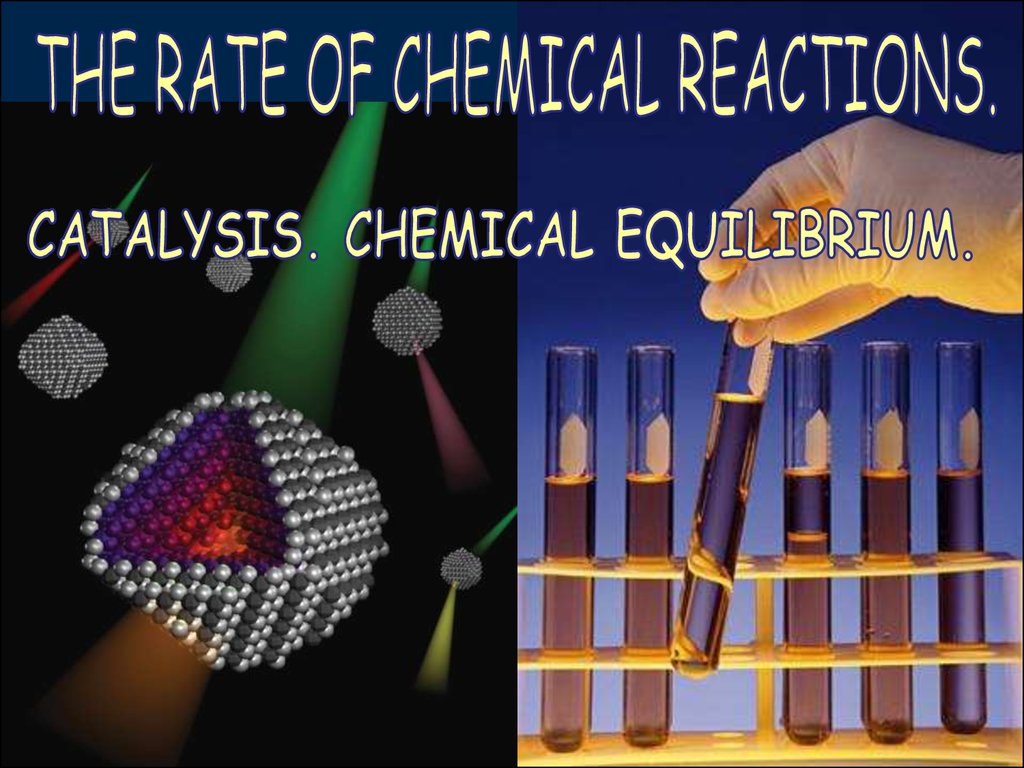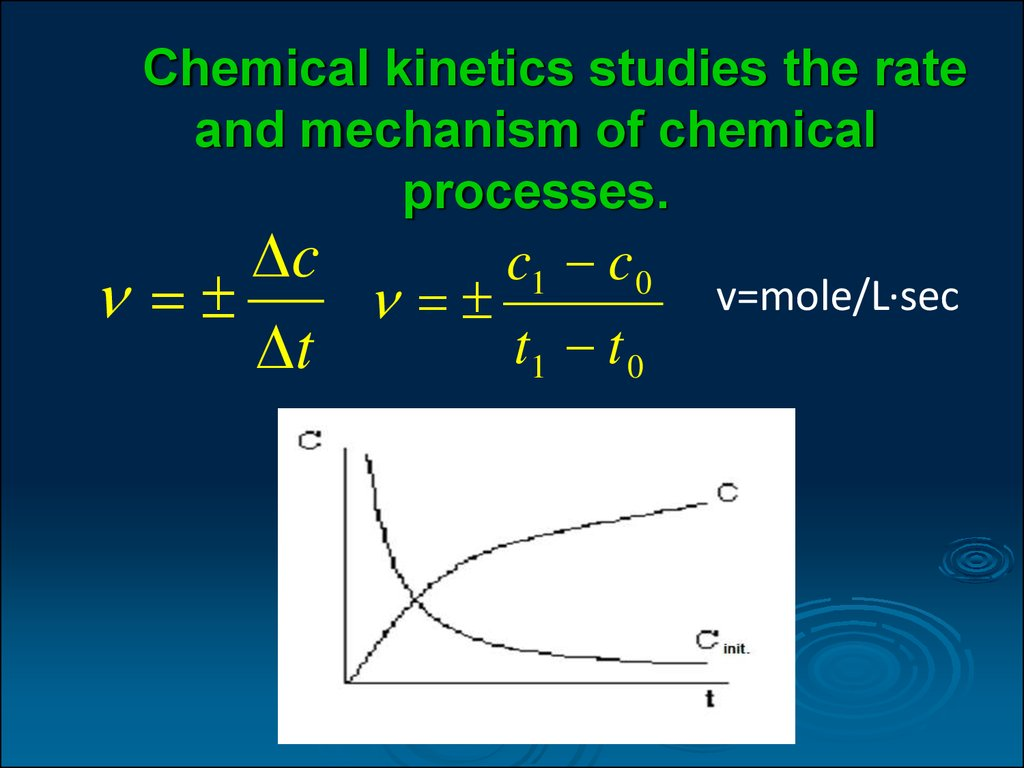# Factors affecting the rate of chemical reaction

## 2.

Chemical kinetics studies the rate
and mechanism of chemical
processes.
c
c1 c 0
t1 t 0
t
v=mole/L∙sec

## 3. Factors affecting the rate of chemical reaction

The chemical reaction rate depends on:
1.The nature of reacting substances:
Н2 + F2 → 2HF
(in the dark, in cold with the explosion)
Н2 + Cl2
2HCl (in the light)
hv
H2 + I2
t 0C
2HI (the reaction is
reversible)

## 4. Factors affecting the rate of chemical reaction

The chemical reaction rate depends
on:
2. The state of the reacting substances.
3. Environment the reaction is proceeding
in.
4. The external conditions.
5. Concentration.

## 5.

The dependence of the chemical
reaction rate on temperature.
J. Van't Hoff formulated a empirical rule: when
temperature rises to 10 K, the rate of most reactions
increases by 2-4 times.
γ=КТ+10/КТ
where γ – the coefficient of the Van't Hoff;
КТ – the reaction rate constant at the initial temperatureТ;
КТ+10 – the reaction rate constant at a temperature of 10
K higher.

## 6.

THE DEPENDENCE OF THE CHEMICAL
REACTION RATE ON TEMPERATURE.
t t
2
t2 t1
10
1
Where Vt2 – rate of a chemical reaction when the temperature
is 10°C higher than the initial speed Vt1;
γ – Van't Hoff’s coefficient ;
t2 – temperature 10°C higher than the initial temperature t1

## 7.

Mass action law
The mass action law: at a constant temperature chemical
reaction rate is proportional to the product of reacting
substances concentrations taken in the power of their
stoichiometric coefficient.
xA yB zAB
k c A cB
x
y
where К – Chemical reaction rate constant, СА and СВ the molar
concentrations of reacting substances, х, y, z - stoichiometric
coefficients.
К=ν,
where СА=СВ=1 mole/L.

## 8.

Mass action law
for heterogeneous reactions
In case of a chemical reaction occurrence at the interface
(gas-liquid, liquid-solid, solid-gas) the surface area of ​the
interface should be taken into account .
xAsol. yB gas zAB
S k c A cB
x
y
where К – Chemical reaction rate constant, S - surface area
of the phase separation, СА and СВ the molar
concentrations of reacting substances, х, y, z stoichiometric coefficients.

## 9.

Kinetic chemical reactions classification
according to the molecularity and order of
reaction
Molecularity of reactions is determined by the number of
molecules participating in the elementary act of interaction.
Most common mono-, bi-and trimolecular reaction.
Decomposition reaction
CaCO 3
CaO+CO 2
The reaction of compound
H 2 + I2
2H I
Oxidation reaction
Hydrolysis
2N O + O 2
C12H22O11 + H2O
(1)
(2)
2NO 2
(3)
2C6H12O6 (4)

## 10.

Kinetic classification of chemical
reactions
Order reaction is defined as the sum of the
degrees of concentration in the kinetic equation.
For example:
1 v=kCCaCO n=1,
2 v=kCH CI
n=2,
3 v=kC2NO CO n=3,
4 CH O=const, v=kCC
order reaction
3
2
2
2
2
12H22
1st order
2nd order
3rd order
O - this is a first
11

## 11.

The rate constant.
The rate constant has an expression and a dimension.
1 Co
1
I _ order _ K ln
sec ,
t C
where _ t time, Сo init . _ concentration,
С final _ concentration _ at _ the _ time _ t
1 1 1
1 L
II _ order _ K ( )
t C Co sec mole
1 1
1 1 L
III _ order _ K 2
2
t 2C
2Co sec mole
2

## 12.

The period of half-transformation
In the kinetics the notion of the period of half-transformation t1/2. is often used
The period of half-transformation is the time during which reacts half
the concentration of initial substances.
ln 2
1
I _ order _ K
sec
t1/ 2
1
1
L
II _ order _ K
t1/ 2 Co sec mole
1 3
1 L
III _ order _ K
t1/ 2 Co sec mole
2

## 13.

Methods for determining the order of
reaction:
The substitution method.
The graphical method.
Differential
method.

## 14.

The activation energy.
A significant increase of the reaction rate as the
temperature increases can be explained by the clash of
active particles with a large reserve of energy. These
include:
- fastest molecules whose kinetic energy Ec ≥ 9,7 kJ /
mol.
- excited molecules.
The energy required for the conversion
of inactive particles in active is called the
activation energy Ea kJ/mol.

## 15.

Arrhenius Equation
K A e
Ea / RT
Ea
ln K ln A
RT
К – the reaction rate constant;
А – a constant value or the total number of collisions;
е – base of the natural logarithm;
R – gas constant;
T – temperature;
Ea – activation energy.

## 16. Catalytic reactions

Catalysis is the process of changing the rate of
reaction by catalysts.
Reactions taking place with the participation of
catalysts called catalytic.
A catalyst is a substance that changes the rate
of a chemical reaction, but it is not spent.
Catalysis has specificity:
H3C
H3C
H2
C
H2
C
OH
[Al2O3]
[Cu]
OH
H2C
CH2
H2O
O
H3C
C
H
H2

## 17. Enzymes

Enzymes are protein molecules able to accelerate
the course of biochemical reactions. Other than
enzymes-proteins there are so-called ribozymes RNA capable of catalysis.

## 18.

The active center is a plot of an enzyme which is
binding, and the transformation of molecules of
substrate.
E - enzyme
P - product
S – substrate
I - inhibitor
[ES] – enzyme-substrate
complex
[EP] – enzyme-product
complex

## 19. Factors affecting the activity of the enzyme

The concentration of the substrate.
In 1913 Michaelis and Menten proposed equation
= max[S]/Km+[S]
Km - Michaelis constant.
A limiting factor of the reaction is the formation of the
enzyme-substrate complex.
Km= the substrate concentration at which the reaction
rate equals to half of the rate to the maximum.

## 20. Specificity of enzymes:

highly specific;
law specific;
nonspecific.
trypsin
Most of the enzymes are highly specific,
since they change only 1 substrate.
Low specific interact with a group of related
substances.
Nonspecific change substances of different
groups.

## 21. The mechanism of action of enzymes

Classic catalysts operate
due to the energy of
activation. Catalysts do
not change G they
reduce the activation
energy. The decrease of
activation energy
increases the number of
molecules able to
overcome the energy
barrier.

## 22. The principle of irreversibility of chemical reactions

to the formation of gaseous substances:
Zn + 2H2SO4 (k) → ZnSO4 + SO2 ↑+ 2H2O
sedimentation:
Ba(NO3)2 + Na2SO4 → BaSO4 ↓+ 2NaNO3
weak electrolyte:
Na2S + 2HCl → 2NaCl + H2S (in solution)

## 23.

Reversible chemical reactions. Equilibrium constant.
Reactions that proceed in opposite directions are called reversible.
V1=K1CH2CI2; V2=K2CHI
2
H2+I2
V1
V2
2HI
At the moment of equilibrium V1=V2, means K1CH2CI2 = K2CHI2
Where К1\К2 = CHI2 \ CH2CI2 =Кр
The equilibrium constant is equal to the ratio of the concentration of
the reaction product to product concentrations of initial substances, taken
in power of stoichiometric coefficient.
For equilibrium processes
0<Кр<
Кр does not dependend on the concentration of substances. Depends on
the nature and temperature.

## 24.

LE CHATELIER'S PRINCIPLE
Shift of the equilibrium based on the principle of Le
Chatelier:
If the system is in a stable equilibrium, external influence
upon changing any of the conditions determining the
equilibrium position of the system will increase the
directions of the process, which weakens the impact of
exposure, and the equilibrium will shift in the same
direction.
1. The increase in the concentration of initial substances
shifts the balance in the direction of increasing the
concentration of the reaction products. And Vice versa.
2. Pressure increase shifts the balance in the direction of
reducing the volume of the system.
3. Temperature influence: temperature increase shifts the
balance in the direction of the process that is accompanied
by absorption the heat.

## 25.

LE CHATELIER'S PRINCIPLE
2СО + О2 = 2СО2; ∆Н<0.
Equilibrium condition : ∆G =0; and ∆G=∆H-T∆S.
G - Gibbs energy (j/mol),
Н – enthalpy (j/mol),
S – entropy (j/mol * K)
The binding of oxygen by hemoglobin to
form oxyhemoglobin occurs according to
the equation:
Hb + O2
HbO2
[ HbO 2 ]

1300
[ Hb ][O2 ]
Increase [O2] leads to the binding O2 and Hb and shift the equilibrium to
the right, i.e. in favour of formation HbO2 and Vice versa.

## 26.

LE CHATELIER'S PRINCIPLE
Acid - base balance of the body is disturbed in violation of the balance between
acid and base:
NaOH + HCl
NaCl + H2O
If the reaction results in the formation of gas, insoluble or poorly soluble
substance, which would leave the scope of the reaction, the balance shifts to the
right.
NaHCO3 + HCl
BaCl2 + Na2SO4
NaCl + H2O + CO2
BaSO4 + 2NaCl
This reaction is used for preparation of radiopaque drug ВаSO4
Ability to use the principle of Le Chatelier's principle allows to predict changes
in the body, caused by external influence.

## 27. The decrease of activation energy is achieved by:

1. Orientation substrates.
2. Theory of steric interactions.
Fischer suggested that the active
center of spatially corresponds to
the substrate molecule. Due to its
spatial specificity of an enzyme
and the substrate are oriented
specifically.

## 28.

3. The theory of
induced correspondence.
Suggested by
Koshland. After the
formation of the enzymesubstrate complex, inside
the enzyme molecule
some conformation
changes can be
observed. They induce
corresponding changes in
the substrate molecule.

## 29.

4. the formation of intermediate complexes.
а) the acid-basic catalysis.
In the enzyme donors protons can be cysteine amino acid
residues, glutamate, aspartate, lysine, gistidine.
Acceptors of protons are the same groups but in the
deprotonated form.
b) covalent catalysis.
During it the substrate or part of it formes stable covalent
bonds with the enzyme molecule.
c) nucleophilic-electrophilic attack

## 30.

PHOTOCHEMICAL
REACTIONS
Photochemical reactions occur with the
absorption of light energy
For example, photosynthesis of the
glucose:
6СО2 + 6Н2О
hv
С6Н12О6 + 6О2

## 31. Mechanisms of chemical reactions

Atoms, molecules, radicals, or ions may participate
in reaction. It’s simple, ion, and radical reaction.
Reactions occurring between the molecules are
called simple : H2 + I2=2HI
H
H
H
H
H
H
I
I
I
I
I
I
2NO + Cl2=2NOCl
Activation energy is 150-450 kJ/mol.

## 32. Mechanisms of chemical reactions

Chain reactions. Radical reactions proceed by a chain mechanism.
Their peculiarity lies in the fact that a primary activation leads act to
transform a huge number of molecules in the raw materials radicals.
For example, the reaction
H2 + Cl2=2HCl
proceeds by a radical chain mechanism by heating or by lighting
light. Due to the absorption of a photon (h ) Cl2 molecule dissociates
into free radicals - chlorine atoms:
Сl2 + h =Сl + * Сl
Atom radical Cl * then reacts with the hydrogen molecule, forming a
molecule of HCl and atom radical * N. Last interacts with a molecule
of Cl2, HCl and atom forms a radical Cl *, etc.
*Сl + Н2=НСl + *Н
*Н + Сl2= НСl + *Сl and etc.
On each absorbed quantum of light there is formed up to 100,000
molecules НСl